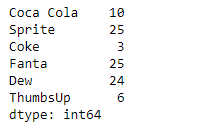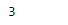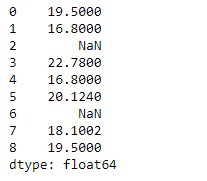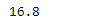GeeksforGeeks App
Open AppBrowser
Continue

# Python | Pandas Series.min()

Pandas series is a One-dimensional ndarray with axis labels. The labels need not be unique but must be a hashable type. The object supports both integer- and label-based indexing and provides a host of methods for performing operations involving the index.

Pandas` Series.min()` function return the mode of the underlying data in the given Series object. This function always returns Series even if only one value is returned.

Syntax: Series.min(axis=None, skipna=None, level=None, numeric_only=None, **kwargs)

Parameter :
axis : Axis for the function to be applied on.
skipna : Exclude NA/null values when computing the result.
level : If the axis is a MultiIndex (hierarchical), count along a particular level, collapsing into a scalar.
numeric_only : Include only float, int, boolean columns.
**kwargs : Additional keyword arguments to be passed to the function.

Returns : min : scalar or Series (if level specified)

Example #1: Use `Series.min()` function to find the minimum value among the underlying data in the given series object.

 `# importing pandas as pd``import` `pandas as pd`` ` `# Creating the Series``sr ``=` `pd.Series([``10``, ``25``, ``3``, ``25``, ``24``, ``6``])`` ` `# Create the Index``index_ ``=` `[``'Coca Cola'``, ``'Sprite'``, ``'Coke'``, ``'Fanta'``, ``'Dew'``, ``'ThumbsUp'``]`` ` `# set the index``sr.index ``=` `index_`` ` `# Print the series``print``(sr)`

Output :Now we will use `Series.min()` function to find the minimum value of the given series object.

 `# return the minimum value in the ``# series object``result ``=` `sr.``min``()`` ` `# Print the result``print``(result)`

Output :As we can see in the output, the `Series.min()` function has successfully returned the minimum value of the given series object.

Example #2: Use `Series.min()` function to find the minimum value among the underlying data in the given series object. The given series object also contains some missing values.

 `# importing pandas as pd``import` `pandas as pd`` ` `# Creating the Series``sr ``=` `pd.Series([``19.5``, ``16.8``, ``None``, ``22.78``, ``16.8``, ``20.124``, ``None``, ``18.1002``, ``19.5``])`` ` `# Print the series``print``(sr)`

Output :Now we will use `Series.min()` function to find the minimum value of the given series object. we are going to skip the missing values while finding the minimum value.

 `# return the minimum value in the ``# series object``result ``=` `sr.``min``(skipna ``=` `True``)`` ` `# Print the result``print``(result)`

Output :As we can see in the output, the `Series.min()` function has successfully returned the minimum value of the given series object.

My Personal Notes arrow_drop_up